Dienes' Logiblocs

Age 5 to 7 Challenge Level:

What is the same and what is different about these two shapes?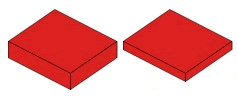What is the same and what is different about these two shapes?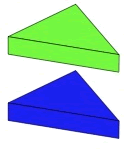What is the same and what is different about these two shapes?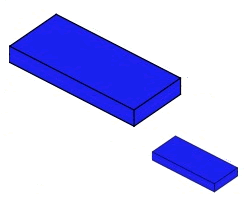What is the same and what is different about these two shapes?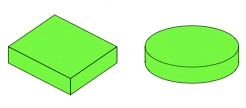Here is a set of shapes: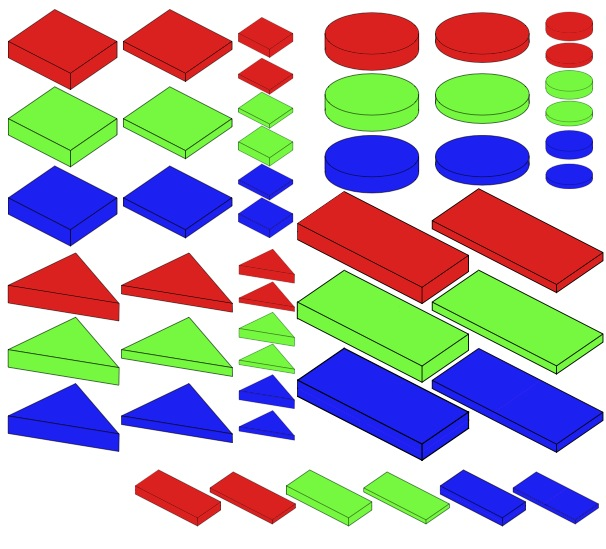What do you notice about them?
Can you describe the shapes?

I could draw a 'difference map' with some shapes. If I join the shapes with just one line it means there is just one difference between the shapes, if I draw two lines there are two differences etc.

For example: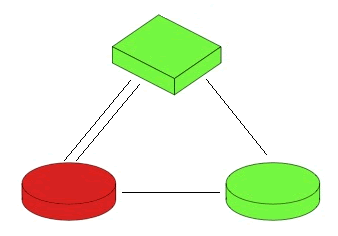Can you see how this works?

I could draw the difference map above like this, where I can put other shapes in the empty boxes: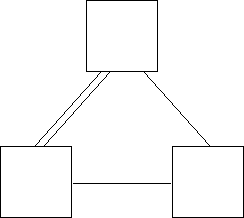Can you fill in the boxes with shapes so that your map uses different shapes to mine?

Can you make a difference map which includes more than three shapes? (It doesn't have to be a closed loop.)

Can you make a difference map which doesn't have any single lines?